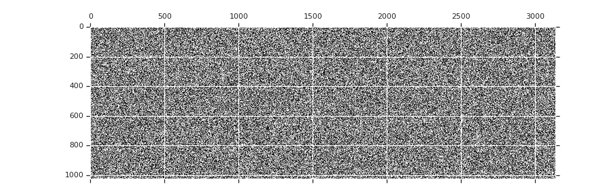# TensorFlow 模型优化工具包 — 剪枝 API

## 权重剪枝

``````import tensorflow_model_optimization as tfmot

model = build_your_model()

pruning_schedule = tfmot.sparsity.keras.PolynomialDecay(

initial_sparsity=0.0, final_sparsity=0.5,

begin_step=2000, end_step=4000)

model_for_pruning = tfmot.sparsity.keras.prune_low_magnitude(model, pruning_schedule=pruning_schedule)

...

model_for_pruning.fit(...)
``````

## 它的工作原理是什么？## 致谢